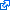# 1 paper accepted to IEEE CSL

Our paper  on the existence and uniqueness of high-voltage solutions of power flow equations in tree networks has been IEEE Control System Letters!K. Dvijotham, E. Mallada, and J. W. Simpson-Porco, “High-Voltage Solution in Radial Power Networks: Existence, Properties, and Equivalent Algorithms,” IEEE Control Systems Letters, vol. 1, iss. 2, pp. 322-327, 2017.

The AC power flow equations describe the steady-state behavior of the power grid. While many algorithms have been developed to compute solutions to the power flow equations, few theoretical results are available characterizing when such solutions exist, or when these algorithms can be guaranteed to converge. In this paper, we derive necessary and sufficient conditions for the existence and uniqueness of a power flow solution in balanced radial distribution networks with homogeneous (uniform R/X ratio) transmission lines. We study three distinct solution methods: fixed point iterations, convex relaxations, and energy functions – we show that the three algorithms successfully find a solution if and only if a solution exists. Moreover, all three algorithms always find the unique high-voltage solution to the power flow equations, the existence of which we formally establish. At this solution, we prove that (i) voltage magnitudes are increasing functions of the reactive power injections, (ii) the solution is a continuous function of the injections, and (iii) the solution is the last one to vanish as the system is loaded past the feasibility boundary.

``````@article{dms2017ieee-csl,
abstract = {The AC power flow equations describe the steady-state behavior of the power grid. While many algorithms have been developed to compute solutions to the power flow equations, few theoretical results are available characterizing when such solutions exist, or when these algorithms can be guaranteed to converge. In this paper, we derive necessary and sufficient conditions for the existence and uniqueness of a power flow solution in balanced radial distribution networks with homogeneous (uniform R/X ratio) transmission lines. We study three distinct solution methods: fixed point iterations, convex relaxations, and energy functions - we show that the three algorithms successfully find a solution if and only if a solution exists. Moreover, all three algorithms always find the unique high-voltage solution to the power flow equations, the existence of which we formally establish. At this solution, we prove that (i) voltage magnitudes are increasing functions of the reactive power injections, (ii) the solution is a continuous function of the injections, and (iii) the solution is the last one to vanish as the system is loaded past the feasibility boundary.},
author = {Dvijotham, Krishnamurthy and Mallada, Enrique and Simpson-Porco, John W.},
doi = {10.1109/LCSYS.2017.2717578},
grants = {1544771},
journal = {IEEE Control Systems Letters},
keywords = {Power Networks; Power Flow Solutions},
month = {10},
number = {2},
pages = {322-327},
title = {High-Voltage Solution in Radial Power Networks: Existence, Properties, and Equivalent Algorithms},